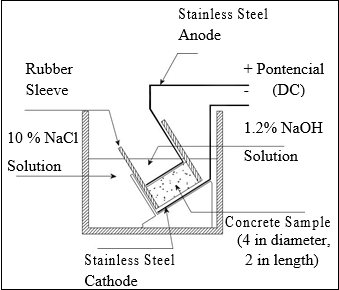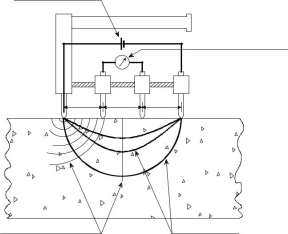Disclaimer: This is an example of a student written essay.

Any scientific information contained within this essay should not be treated as fact, this content is to be used for educational purposes only and may contain factual inaccuracies or be out of date.

# Rapid Migration Test

 ✅ Paper Type: Free Essay ✅ Subject: Engineering ✅ Wordcount: 1292 words ✅ Published: 30th Aug 2017

The RMT involves subjecting a 4-inch (102- mm) diameter by 2- inch (51-mm) thick saturated samples to an external electrical potential to force chlorides ions to migrate into the specimens (NT BUILD 492 1999). To account for varying concrete resistances, the initial current flow through the specimen is measured and the applied voltage is adjusted accordingly. The samples are fit into silicone rubber sleeves where one of the sides of the specimens is immersed in a 0.3 N NaOH (1.2% NaOH) solution and the other side to a 10 percent NaCl solution. After a specified duration, the samples are removed and axially split into two pieces. A depth of chloride penetration is determined in one half of the specimen using a colorimetric technique; spraying silver nitrate solution on the freshly cut surface.The originally proposed method called for the concrete sample to be exposed to a voltage gradient for 8 hours, after which the specimen is sliced and sprayed with an indicator for chlorides, AgNO3 to determine the depth of chloride penetration. This time period makes the procedure difficult to fit into a normal working day of a laboratory. Consequently, Tang and Nilsson revised their method to use varying voltages and test durations depending upon the initial current measured (NTBuild 492). This improved test was standardized as a Nordtest procedure.

If you need assistance with writing your essay, our professional essay writing service is here to help!

The standardized method NTBuild 492 still presented further problems. The most critical is the extended time duration of the test (as long as 4 days in some cases) and the wide range of applied voltage that must be used. A simplified testing protocol was developed in which the effect of several different voltages and test durations were evaluated (Hooton, Thomas and Stanish 2001). Based on the results of their research, a fixed test duration of 18 hours was selected, with a varying applied voltage. The voltage selected for the test is based on the initial current values for that sample under a 60-volt potential. The new proposed voltage values were selected to avoid chloride breakthrough that would occasionally occur in the NTBuild 492 procedure

2.2.6  SURFACE RESISTIVITY TEST USING THE FOUR-POINT WENNER PROBE (FM 5-578)

Concrete conductivity is fundamentally related to the permeability of fluids and the diffusivity of ions through a porous material (Whiting and Mohamad 2003). As a result, the electrical resistivity can be used as an indirect measure of the ease in which chlorides ions can penetrate concrete (Hooton, Thomas and Stanish 2001). The resistivity of a saturated porous medium, such as concrete, is mainly measured by the conductivity through its pore solution (Streicher and Alexander 1995).

Two procedures have been developed to determine the electrical resistivity of concrete. The first method involves passing a direct current through a concrete specimen placed between two electrodes. The concrete resistance between the two electrodes is measured. The actual resistance measured by this method can be reduced by an unknown amount due to polarization at the probe contact interface. The second method solves the polarization problem by passing an alternating current (AC) through the sample. A convenient tool to measure using this method is the four -point Wenner Probe resistivity meter (Hooton, Thomas and Stanish 2001). The set up utilizes four equally spaced surface contacts, where a small alternating current is passed through the concrete sample between the outer pair of contacts. A digital voltmeter is used to measure the potential difference between the two inner electrodes, obtaining the resistance from the ratio of voltage to current (see Figure 5). This resistance is then used to calculate resistivity of the section. The resistivity ρ of a prismatic section of length L and section area A is given by:

ρ= A.RL

where R is the resistance of the specimen calculated by dividing the potential V by the applied current I.

The resistivity ρ for a concrete cylinder can be calculated by the following formula:

 π .d 2 1 V ⋅ ρ = 4 L I

where d is the cylinder diameter and L its length (Morris, Moreno and Sagües 1996). Assuming that the concrete cylinder has homogeneous semi-infinite geometry (the

dimensions of the element are large in comparison of the probe spacing), and the probe depth is far less than the probe spacing, the concrete cylinder resistivity ρ is given by:

 V ρ =(2.π .a)⋅ I

where a is the electrode spacing (see Figure 5). The non-destructive nature, speed, and ease of use make the Wenner Probe technique a promising alternative test to characterize concrete permeability.Current Applied (I) Potential Measured Concrete Surface a a a (V) to be Tested Equipotential lines Current Flow LinesFigure 5. Four-point Wenner Probe Test Setup.

Results from Wenner Probe testing can vary significantly if the degree of saturation or conductivity of the concrete is inconsistent. Techniques to achieve more uniform saturation, such as vacuum saturation or submerging in water overnight, can be performed in the laboratory. However, the laboratory pre-saturation procedure still presents some inconsistencies. The known conductivity of the added solution changes when mixed with the ions (mainly alkali hydroxides) still present in the concrete pores after the drying process (Hooton, Thomas and Stanish 2001). To overcome this problem, Streicher and Alexander (1995) suggested the use of a high conductivity solution, for example 5 M NaCl, to saturate the sample so that the change in conductivity from the ions remaining in the concrete is insignificant.

View all

## DMCA / Removal Request

If you are the original writer of this essay and no longer wish to have your work published on UKEssays.com then please:

Related Services

Prices from

£126

Approximate costs for:

• 1000 words
• 7 day deliveryHumanity University

Dedicated to your worth and value as a human being!

Related Lectures